Courses

# Test: Problems On AC And DC Analysis

## 10 Questions MCQ Test Electronic Devices | Test: Problems On AC And DC Analysis

Description
This mock test of Test: Problems On AC And DC Analysis for Electrical Engineering (EE) helps you for every Electrical Engineering (EE) entrance exam. This contains 10 Multiple Choice Questions for Electrical Engineering (EE) Test: Problems On AC And DC Analysis (mcq) to study with solutions a complete question bank. The solved questions answers in this Test: Problems On AC And DC Analysis quiz give you a good mix of easy questions and tough questions. Electrical Engineering (EE) students definitely take this Test: Problems On AC And DC Analysis exercise for a better result in the exam. You can find other Test: Problems On AC And DC Analysis extra questions, long questions & short questions for Electrical Engineering (EE) on EduRev as well by searching above.
QUESTION: 1

### In the circuit, transistor has β =60, VBE=0.7V. Find the collector to emitter voltage drop hoe.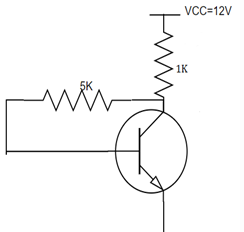Solution:

We know, IC=(VCC-VBE)/RB
By putting the values, we have IC=5.9mA. IE=IC/α. So, IE=5.99mA.
hoe= VCC-RC(IC+IB). We have hoe=6V.

QUESTION: 2

### For the circuit shown, find the quiescent point.​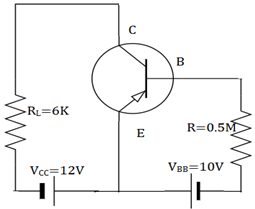Solution:

We know, hoe=12V
(IC)SAT =VCC/RL=12/6K=2mA. IB=10V/0.5M=20µA. IC= βIB=1mA. I
hoe=VCC-ICRL=12-1*6=6V. So, quiescent point is (6V, 1mA).

QUESTION: 3

### For the circuit shown, find the quiescent point.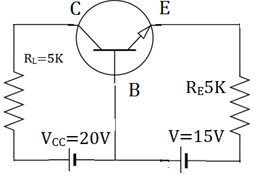Solution:

We know, IE=VEE/RE=10/5kΩ=2mA
IC=α IE =IE =2mA
VCB=VCC-ICRL=20-10=10V. So, quiescent point is (10V, 2mA).

QUESTION: 4

In the circuit shown below, β =100 and VBE=0.7V. The Zener diode has a breakdown voltage of 6V. Find the operating point.​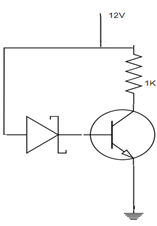Solution:

We know, by KVL -12+(IC+IB)1K+6+VBE=0
We have IE=5.3. IC= αIE=5.24mA. From another loop, -12+IEIK+VBE=0
We have, hoe=12-5.3m*1000=6.7V. Hence the Q point is (6.7V, 5.3mA).

QUESTION: 5

10. When the β value is large for a given transistor, the IC and hoe values are given by_________

Solution:

The base current IB is zero when β value is large. So, the hoe changes to VCC-RCIC. The collector current IC is changed to (VCC-VBE)/RB from β(VCC-VBE)/(1+ β)RE+ RB.

QUESTION: 6

For the circuit shown, find the quiescent point.​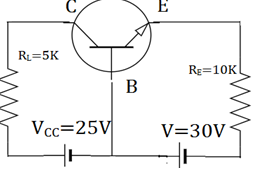Solution:

We know, IE=VEE/RE=30/10kΩ=3mA
IC=α IE =IE =3mA
VCB=VCC-ICRL=25-15=10V. So, quiescent point is (10V, 3mA).

QUESTION: 7

The PNP transistor when used for switching the power, then it is called_________

Solution:

Sometimes DC current gain of a bipolar transistor is too low to directly switch the load current or voltage, so multiple switching transistors is used. The load is connected to ground and the transistor switches the power to it.

QUESTION: 8

In which of the regions, the small capacitors are open circuited?

Solution:

In low frequency region, the small capacitors are open circuited and large capacitors are in active state. In high frequency region, the large capacitors are short circuited and small capacitors are active.

QUESTION: 9

In mid frequency region, the large capacitors are short and small capacitors are open circuited. What happens to the RC coupled circuit?

Solution:

In the mid frequency region, the whole circuit is resistive because the large capacitors are short and small capacitors are open circuited. The gain is constant in this region. So, the circuit is frequency blind as the gain is constant in this region.

QUESTION: 10

In low frequency region, the gain_________

Solution:

At very low frequency, the gain decreases due to the coupling capacitor. When the frequency is decreased, the reactance of the circuit increases and the drop across the coupling capacitor increases. The gain is therefore decreased as the output decreased.